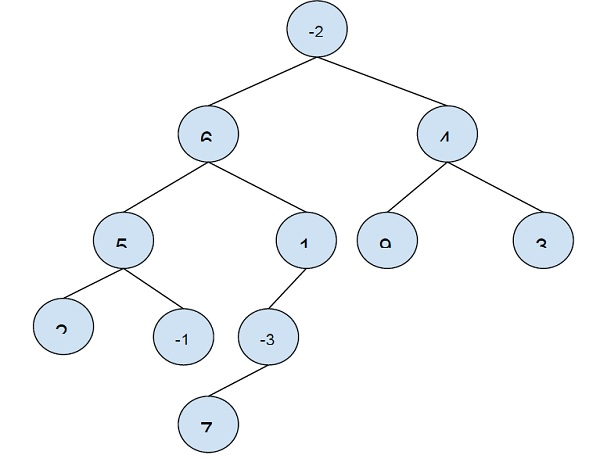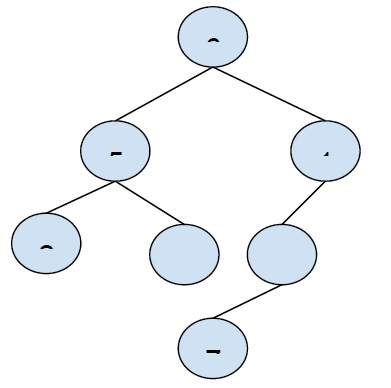# Maximum Path Sum in a Binary Tree in C++

In this problem, we are given a binary tree with each node containing a value. Our task is to create a program to find the maximum path sum between two leaves of a binary tree.

Here, we have to find the path form one leaf node to another leaf node that will provide the maximum sum of values. This maximum sum path can/cannot include the root node.

Binary Tree is a tree data structure in which each node can have a maximum of two child nodes. These are called a left child and right child.

ExampleLet’s take an example to understand the problem −

Input − //binary tree…

Output − 24

Explanation − The path from leaf node − 2 to 9 will give the maximum sum which is (2 + 5 + 6 -2 + 4 + 9 ) = 24

To solve this problem, we will do tree traversal and store the maximum sum for the left sub-tree/ right subtree for the current node. Also, we will find the maximum path between two leaf nodes so far.

For, every node we will find the maximum possible leaf to leaf path for its subtrees. And then compare it with the overall maximum path and store the maximum of both the values in the global maximum path sum value.

Let’s see this solution from our example to understand the solution better −

Global maximum sum = 6 (for path 2→5→-1)

Now we will check to take 6 as root nodes.In left subtree −

The Sum of the path till leaf nodes is 7 and 4.

Maximum is 7 (for path 5→2).

In the right subtree −

Sum of path till leaf nodes is 5 for the path (1rarr;-3rarr;7) which is one possible way.

No, the sum of the path between leaf nodes is −

Maximum sum for leaf to root(6) in left subtree + root + Maximum sum for leaf to root(6) in right subtree = 7 + 6 + 5 = 18.

Comparing with global maximum path sum(6), New global maximum path sum = 18.

## Example

Program to find the maximum path sum between two leaf nodes −

Live Demo

#include <bits/stdc++.h>
using namespace std;
struct Node{
int data;
struct Node* left, *right;
};
struct Node* insertNode(int data){
struct Node* node = new(struct Node);
node->data = data;
node->left = node->right = NULL;
return (node);
}
int max(int a, int b)
{ return (a >= b)? a: b; }
int maxPathSumLeaf(struct Node *root, int &maxSum){
if (root==NULL) return 0;
if (!root->left && !root->right) return root->data;
int leftSubTree = maxPathSumLeaf(root->left, maxSum);
int rightSubTree = maxPathSumLeaf(root->right, maxSum);
if (root->left && root->right){
maxSum = max(maxSum, leftSubTree + rightSubTree + root->data);
return max(leftSubTree, rightSubTree) + root->data;
}
return (!root->left)? rightSubTree + root->data: leftSubTree + root->data;
}
int main(){
struct Node *root = insertNode(-2);
root->left = insertNode(6);
root->right = insertNode(4);
root->left->left = insertNode(5);
root->left->right = insertNode(1);
root->left->left->left = insertNode(2);
root->left->left->right = insertNode(-1);
root->left->right->left = insertNode(-3);
root->left->right->left->left = insertNode(7);
root->right->left = insertNode(9);
root->right->right = insertNode(3);
int maxSum = INT_MIN;
maxPathSumLeaf(root, maxSum);
cout<<"Max pathSum of between two leaf nodes for the given binary tree is "<<maxSum;
return 0;
}

## Output

Max pathSum of between two leaf nodes for the given binary tree is 24